• r语言数据可视化案例
千次阅读
2022-01-10 17:29:07

# 条形图、饼图、treemap及其局部调整

# 条形图、饼图、树形图及其局部调整
library(tidyverse)
mtcars %>%
mutate(cyl = as_factor(cyl)) %>%
ggplot(aes(x = cyl)) +
geom_bar()

mtcars %>%
mutate(cyl = as_factor(cyl)) %>%
ggplot(aes(y = cyl)) +
geom_bar()

library(janitor)
mtcars_cyl <- mtcars %>%
tabyl(cyl)

mtcars_cyl %>%
mutate(cyl = as_factor(cyl)) %>%
ggplot(aes(x = reorder(cyl, -n),
y = n)) +
geom_col(fill = "blue") +
geom_text(aes(label = n),
vjust = -0.5) +
labs(x = "cyl",
y = "Frequency",
title = "mtcars by cyl") +
theme(plot.title = element_text(hjust = 0.5)) +
theme_bw()

library(scales)
mtcars_cyl %>%
mutate(cyl = as_factor(cyl),
plabel = percent(percent)) %>%
ggplot(aes(x = reorder(cyl, -n),
y = percent)) +
geom_r语言
更多相关内容
• R语言数据可视化案例（世界杯球员信息数据可视化）世界杯球员案例数据预处理 世界杯球员案例 在 faraway 包中包含一个名为 worldcup 的数据集（加载 faraway 包后，可通过代码“head(worldcup)”查看数据的前 5 行，...

# 世界杯球员案例

在 faraway 包中包含一个名为 worldcup 的数据集（加载 faraway 包后，可通过代码“head(worldcup)”查看数据的前 5 行，该数据集是 2010 年世界杯上球员的信息数据，每个变量所表示的信息如下：
Team： 国家
Position：位置，包括后卫、前锋、守门员、中场
Time： 上场总时间
Shots： 射门的次数
Passes：传球次数
Tackles：铲球次数
Saves： 救球次数

## 数据预处理

数据概况：

> head(world)
Team   Position Time Shots Passes Tackles Saves
Abdoun      Algeria Midfielder   16     0      6       0     0
Abe           Japan Midfielder  351     0    101      14     0
Abidal       France   Defender  180     0     91       6     0
Abou Diaby   France Midfielder  270     1    111       5     0
Aboubakar  Cameroon    Forward   46     2     16       0     0
Abreu       Uruguay    Forward   72     0     15       0     0


该数据集中，数据集包含595个样本，共有7个特征。其中Team，Postition是分类变量，后面的都是数值型变量，其中行名是球员名字。

1. 导入需要的包和数据集 ，导入需要的包和数据集；
library(faraway)
library(ggplot2)
attach(worldcup)
?worldcup
world=worldcup

1. 上场时间和传球数量的关系
#给散点图变成以颜色做区分位置队伍
p <- ggplot(data=world, mapping=aes(x=world$Time, y=world$Passes, shape=world$Position, colour=world$Team))
p+geom_point()


运行结果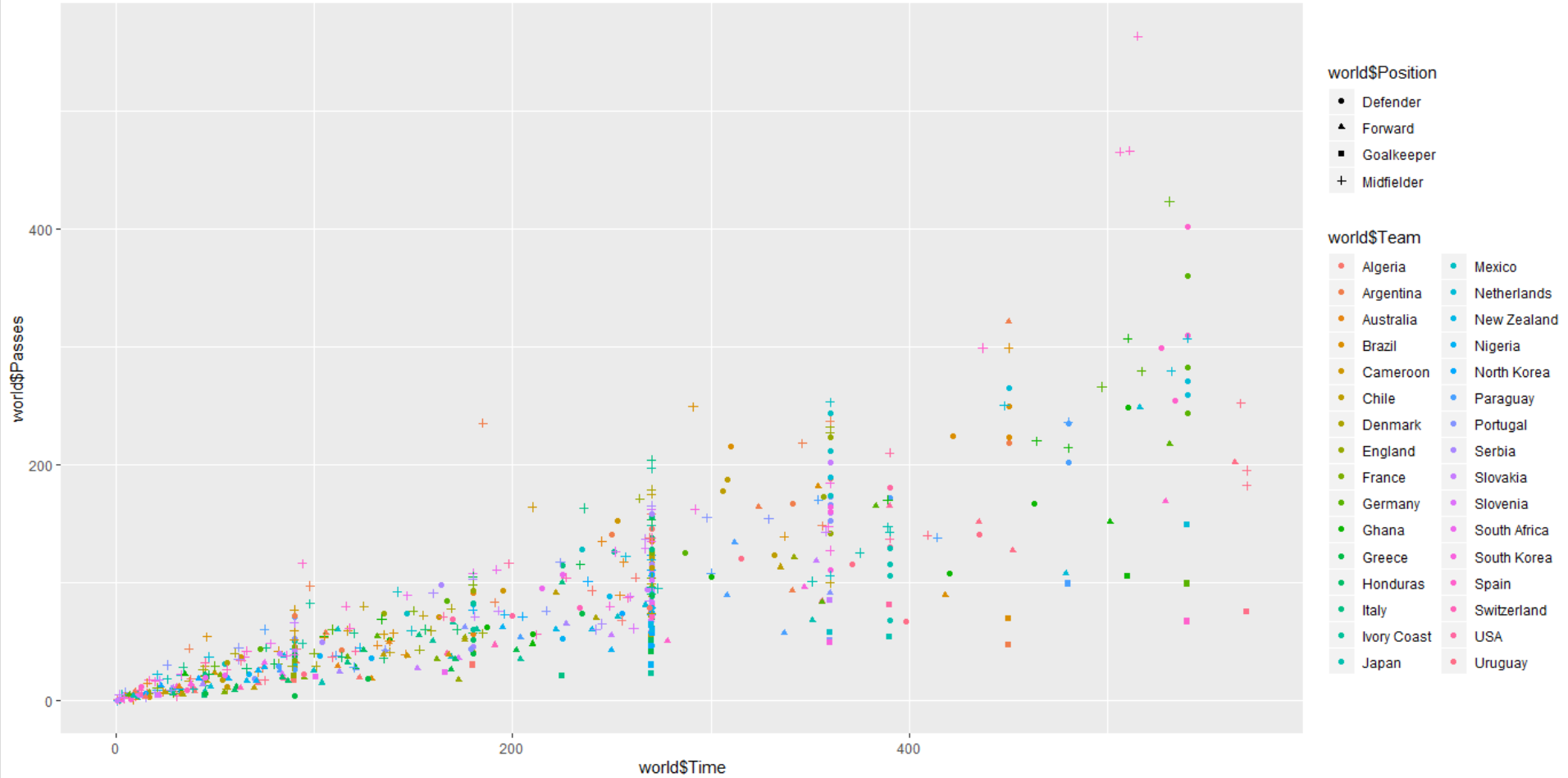由图形可看出，各球员上场时间多集中于 400 以内，传球次数也对应集中于 200以内，其中 Midfifielder 位置的上场时间和传球次数较为出众。同时一定程度上，上场时间越长，传球数越大。
3. 不同位置射门次数直方图

#不同位置射门次数直方图
ggplot(world)+geom_histogram(aes(x=world$Shots)) ggplot(world)+geom_histogram(aes(x=world$Shots, fill=world$Position))  运行结果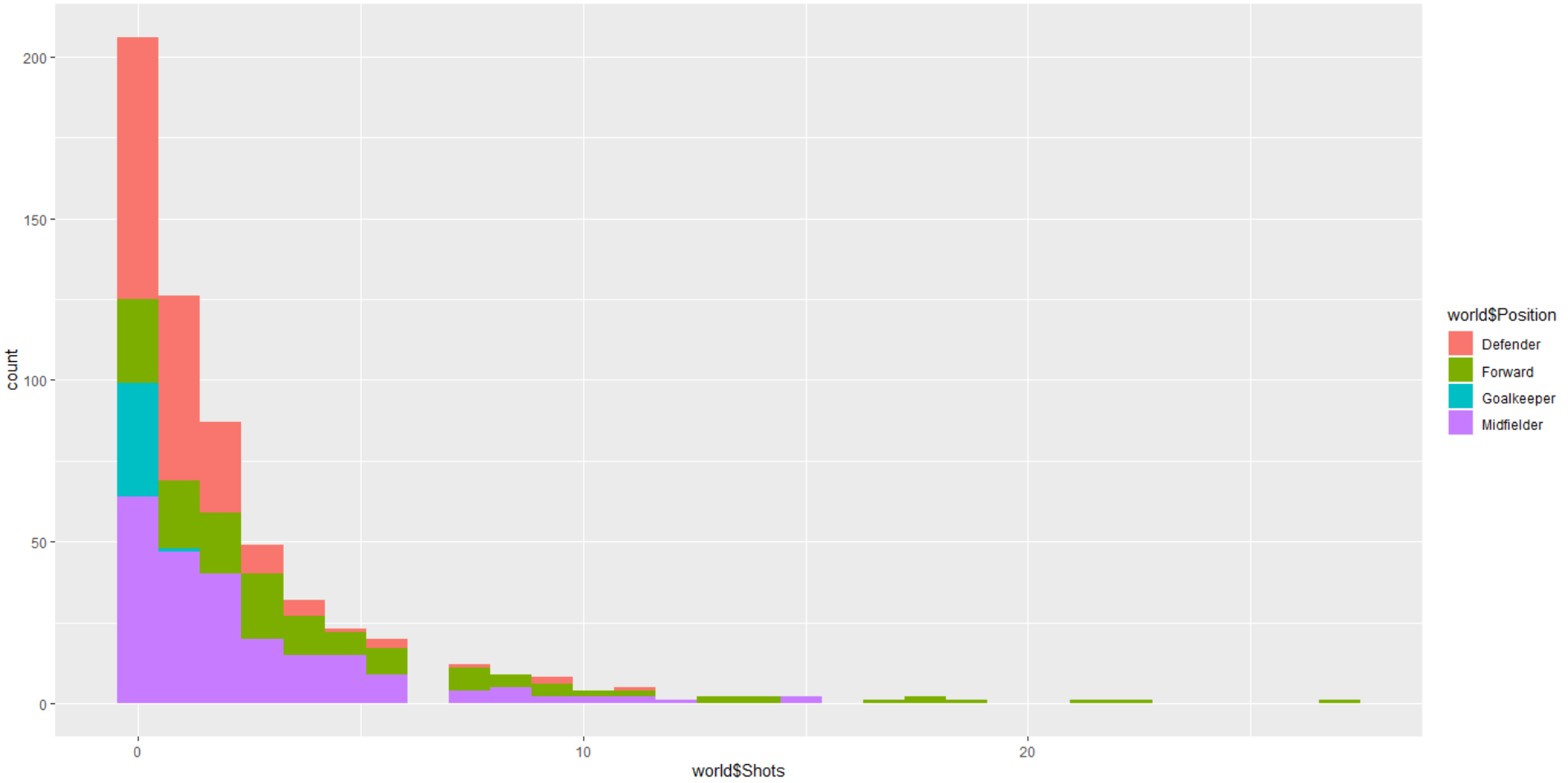由图可发现，大部分球员射门次数集中于 5 个以内，其中 Defender 射门次数较少，而 Forward 射门表现较为优异，符合常理。 4. 不同队伍射门次数直方图 #不同队伍射门次数直方图 ggplot(world)+geom_histogram(aes(x=world$Shots, fill=world$Team)) ggplot(world)+geom_histogram(aes(x=world$Shots, fill=world$Team), position="dodge")  运行结果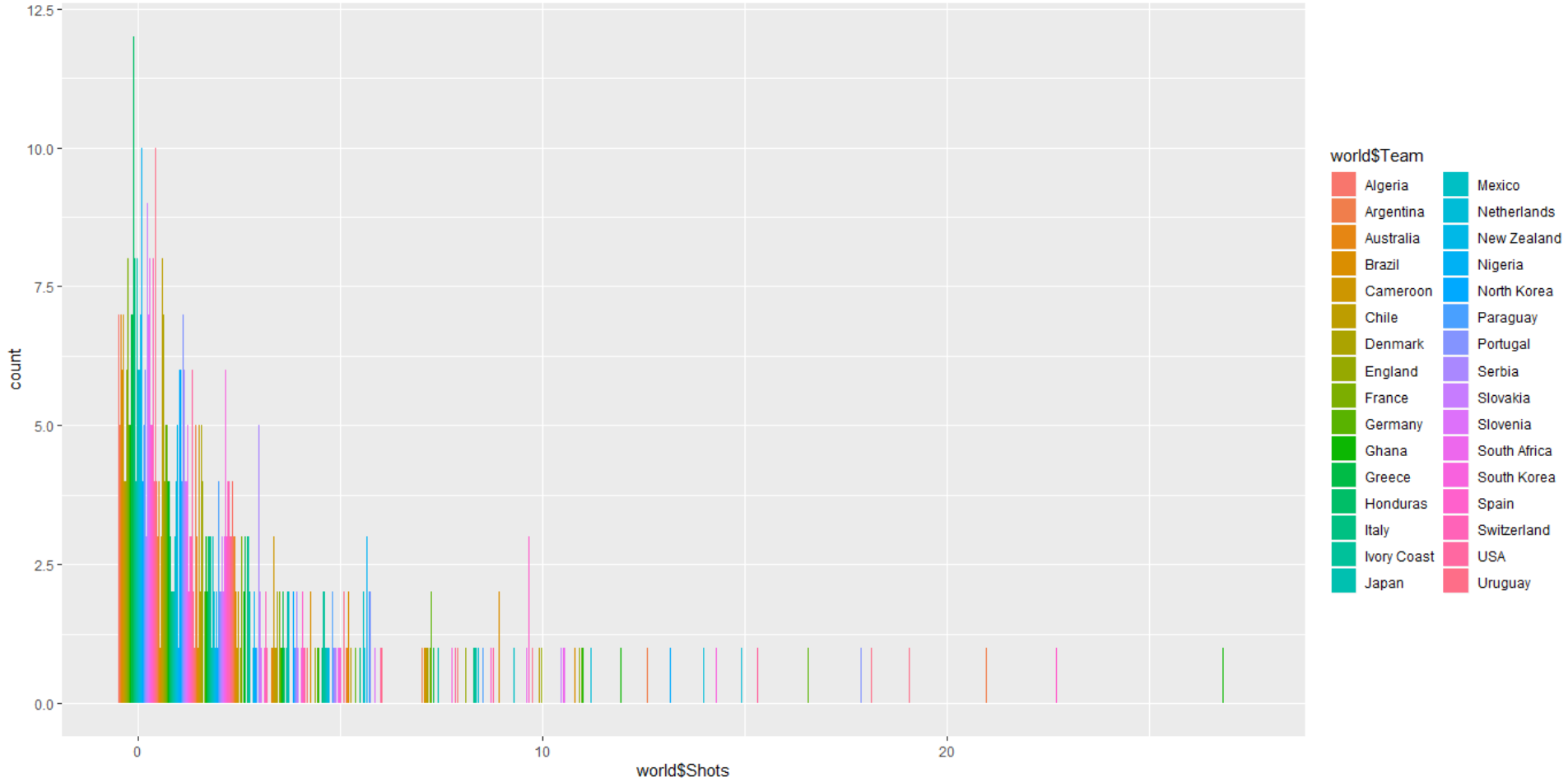5. 不同队伍射门次数直方图 #箱型图 ggplot(world)+geom_boxplot(aes(x=world$Team, y=world$Shots,fill=world$Team))+coord_flip()#不同队伍射门次数


运行结果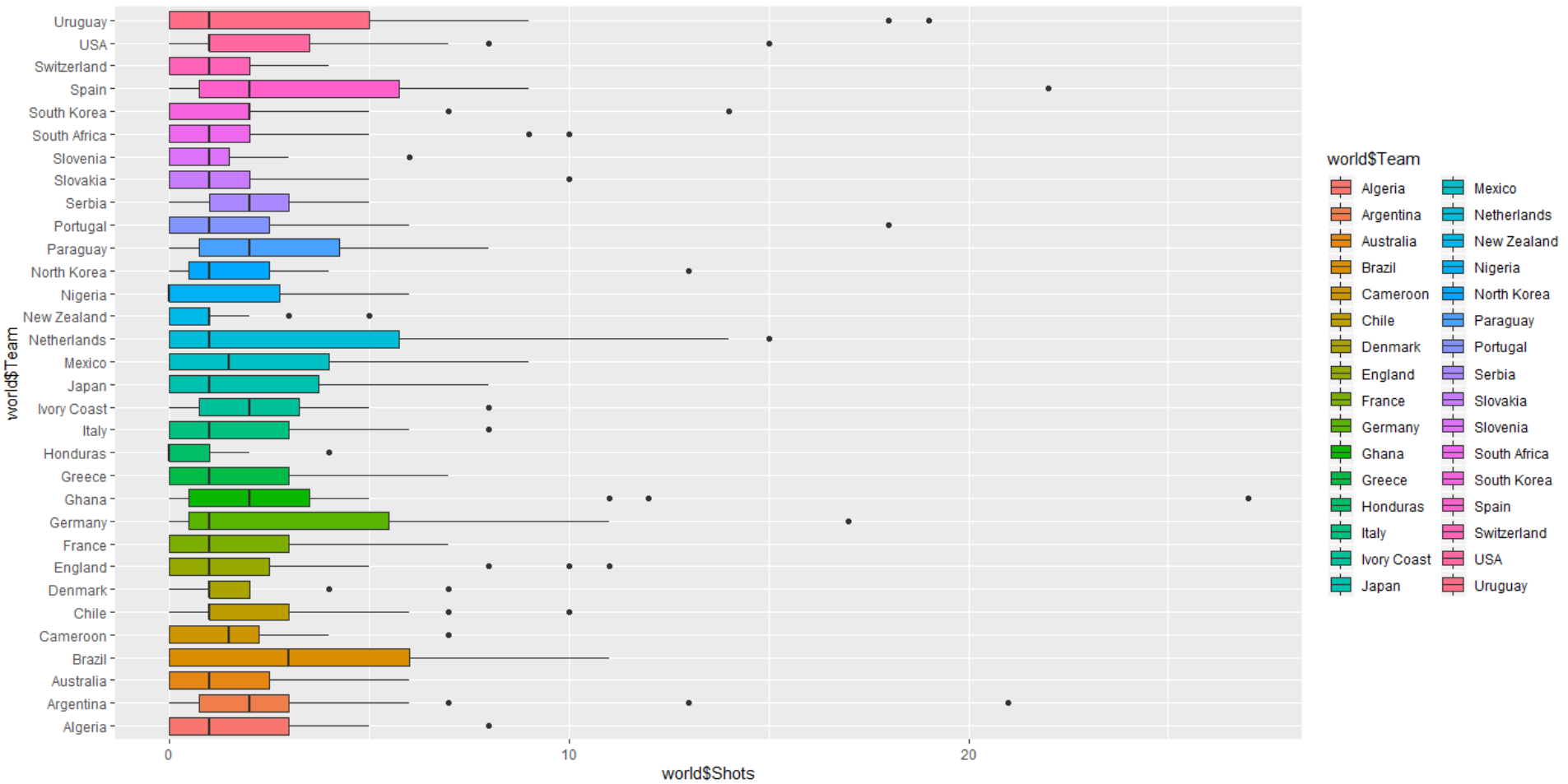由图可知 Brazil 队射门次数平均较高，但无极端异常高的值，可推测其与队伍战术风格有关，以进攻为主，但各队员水平相近，表现稳定，而 Ghana 等队虽然整体平均还行，但是有一个极端高值，可推测是有球员表现尤为优异，带动队伍射门进攻。
6. 绘制球员分布国家地图可视化

n=rep(1,595)#设定重复的1
world=cbind(world,n)
number<-aggregate(x = world$n, by= list(world$Team), FUN = sum)#计算各个国家球员数
colnames(number)<-c("nation","number")
library(map)
library(REmap)
result <- remapC(number,
maptype = 'world',
color = 'red',
theme = get_theme('Sky'),
maxdata = 20,
mindata = 14)
print(result)


运行结果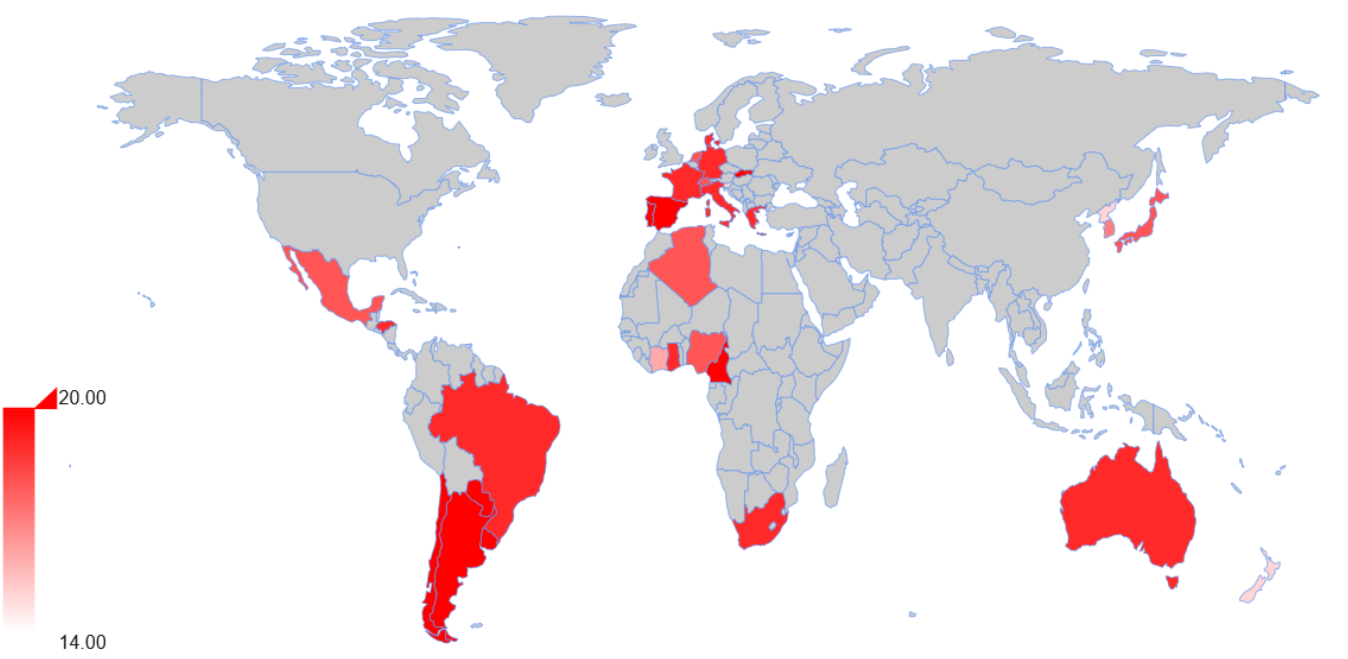可通过地理信息数据发现，球队主要来源于西半球，各队伍成员数量较为相近，无过于显著的差异。
其中，安装REmap时，常规方式安装会报错，可利用下面方式解决：

install.packages("devtools")
library(usethis)
library(devtools)
install_github("Lchiffon/REmap")
library(REmap)

1. 绘制球员人数分布直方图
#分布直方图
par(mai=c(0.9, 0.9, 0.6, 0.3)) #图形边缘空白(边距)的宽度
hist(number$number, col="lightblue", border="red", labels = TRUE,ylim=c(0, 20),main="运动会各队总人数分布")  运行结果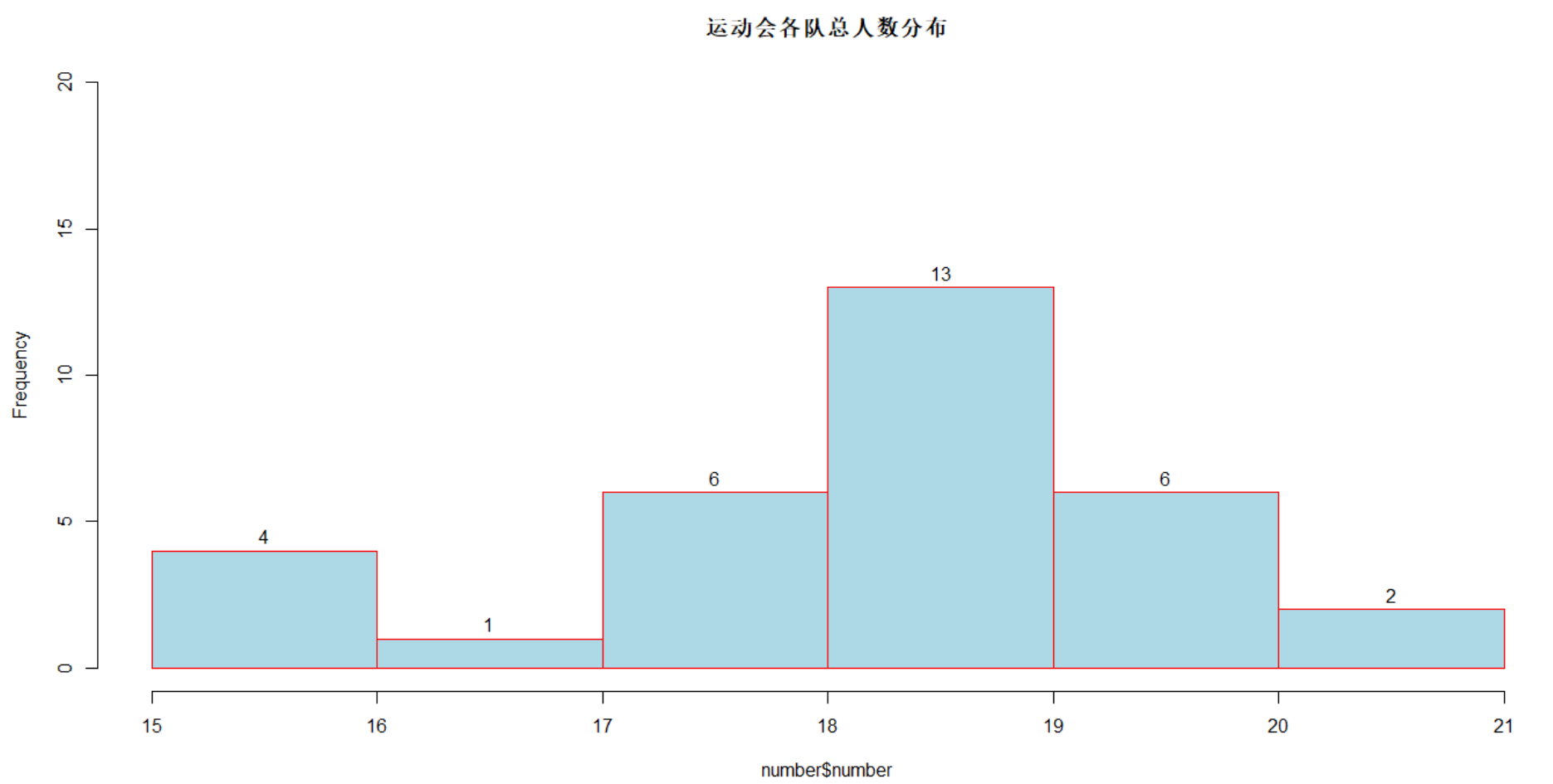可发现人数分布服从近似正态分布。 8. 对不同位置人员进行上场时间与射门次数的分析 #对不同位置人员进行上场时间与射门次数的分析 ggplot(worldcup,mapping = aes(x=Time,y=Shots,colour = Position))+geom_point()  运行结果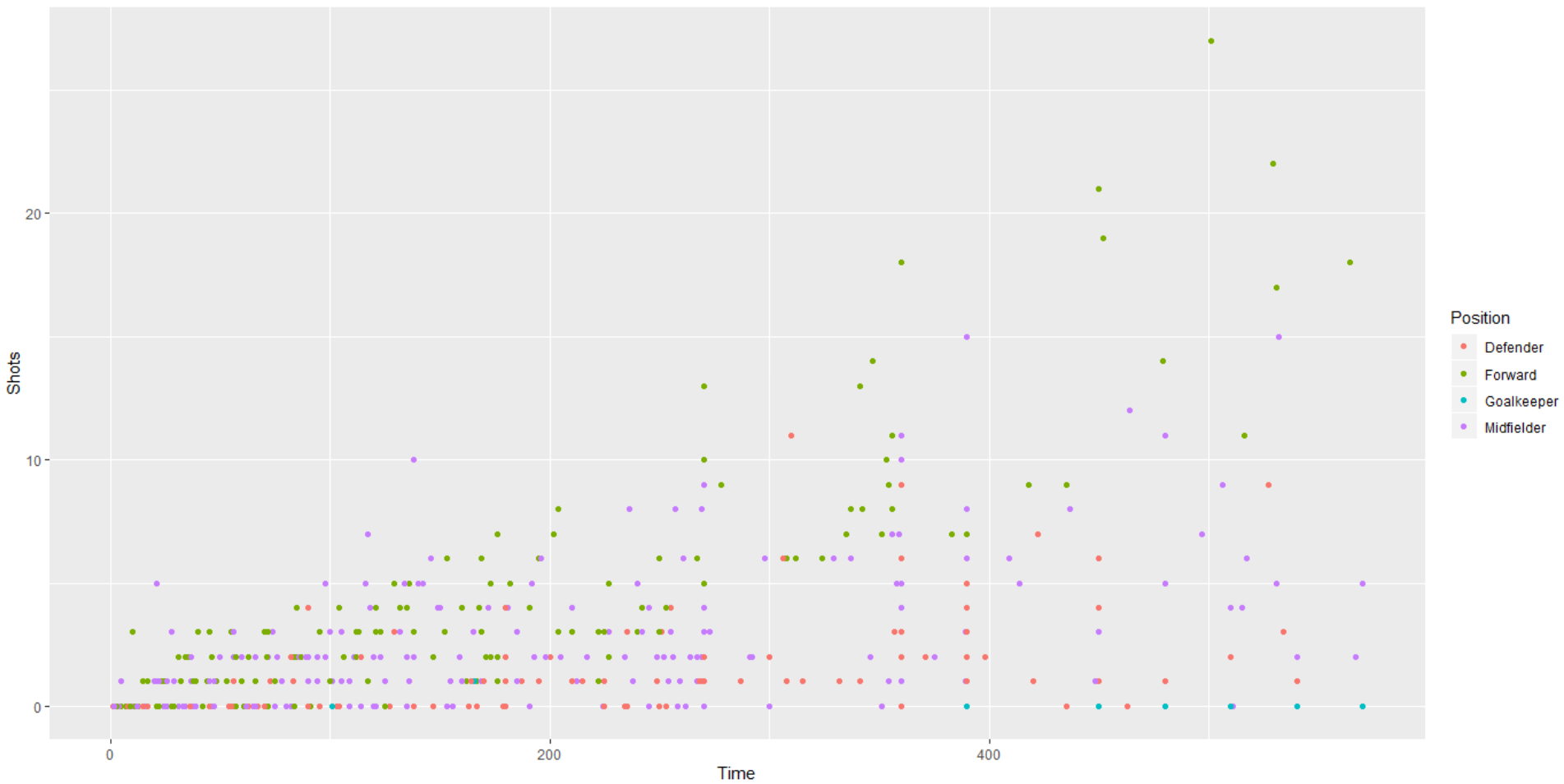由图可发现，Forward 的射门次数都较高于其他位置，符合常理。 9. 国家与射门次数之间的关系 #国家与射门次数之间的关系 ggplot(world,aes(x=world$Team,y=world$Shots,fill=world$Team))+
theme_bw(base_family="STKaiti")+
geom_bar(aes(fill=rownames(worldcup)),stat="identity",show.legend=F)+
coord_flip()


运行结果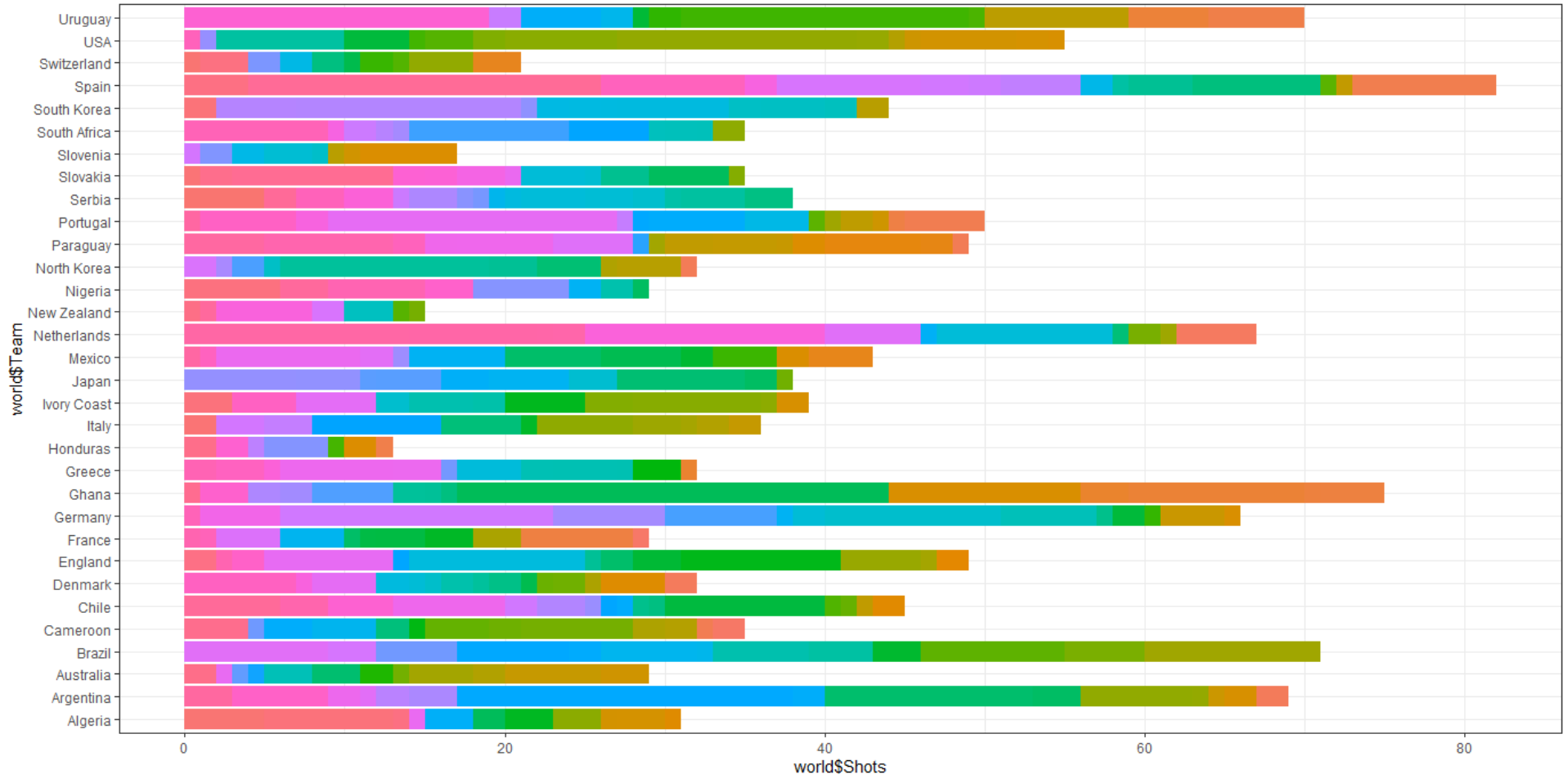从整体来看，Spain 总的射门次数最多，可看出在比赛中处在上风。Honduras 较少处于劣势。
10. 每个运动员上场时间及射门次数关系

library(treemap);
library(dplyr)
plays<-as.data.frame(rownames(world))#每个球员上场时间
plays<-cbind(plays,world$Time,world$Shots)#球员对应上场时间及射门
colnames(plays)<-c("player","time","shots")
treemap(plays,index = c("player"),vSize = "time", vColor= "shots",type="value",palette="RdYlGn",
title = "每个运动员上场时间及射门次数关系",fontfamily.title = "STKaiti",
title.legend= "数量",fontfamily.legend="STKaiti")


运行结果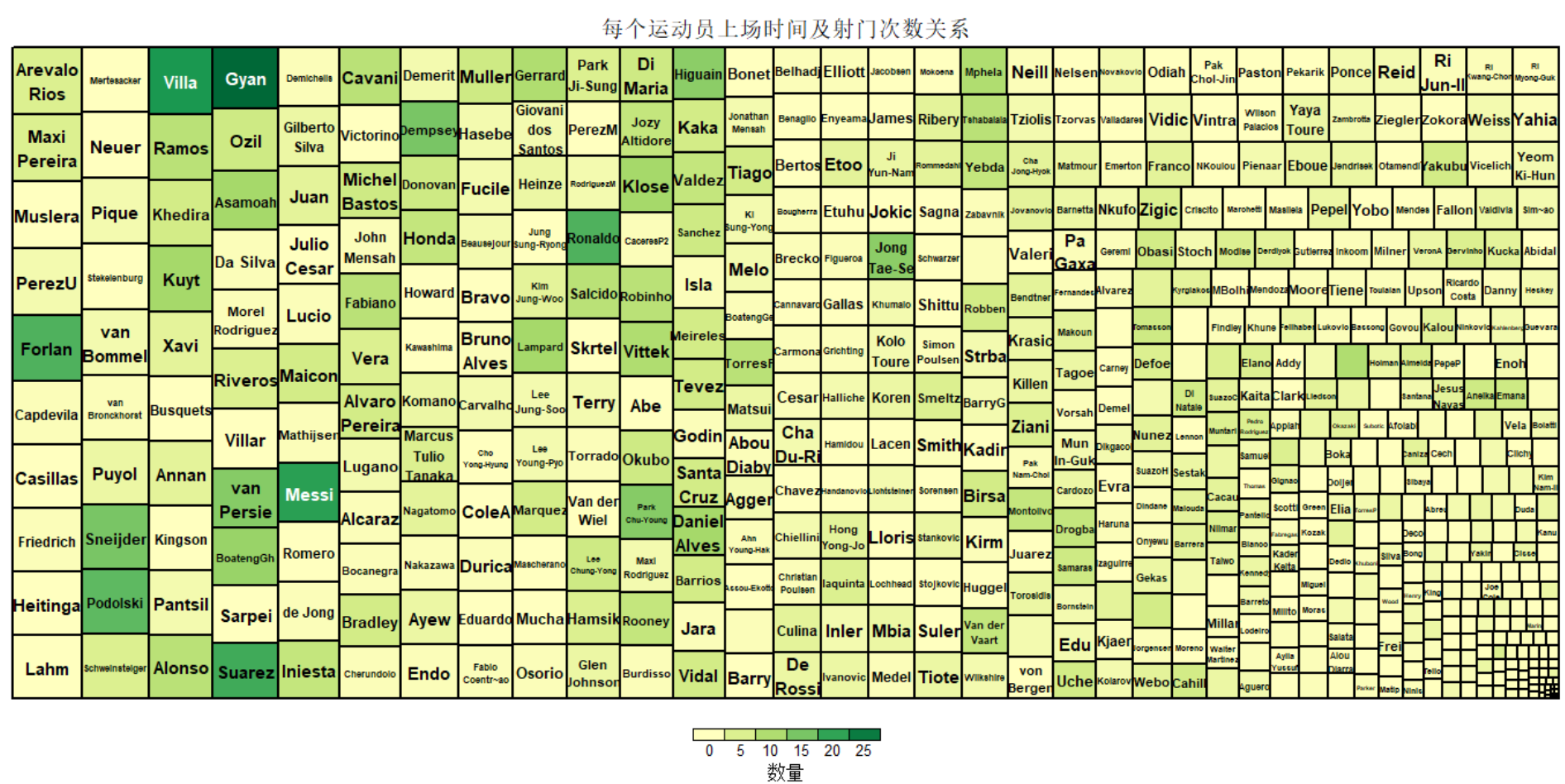图形宽度代表上场时间，颜色深浅代表射门次数。可发现 Gyan,Villa 球员上场时间多，射门次数也多，是团队核心成员，而 Jong Tae-Se 上场时间中等，但射门次数较多，是很有潜力的成员。通过这个图寻找核心成员，同时挖掘潜力成员。
11. 球员上场时间分布图

#球员上场时间分布图
ggplot(worldcup,aes(x=reorder(rownames(worldcup),Time),y=Time))+
theme_bw(base_family="STKaiti")+
geom_bar(aes(fill=Time),stat="identity",show.legend = F)+
labs(x="球员",y="各个球员上场总时间分布",title="上场时间")+
theme(axis.text.x =element_text(vjust = 0.5),plot.title=element_text(hjust=0.5))


运行结果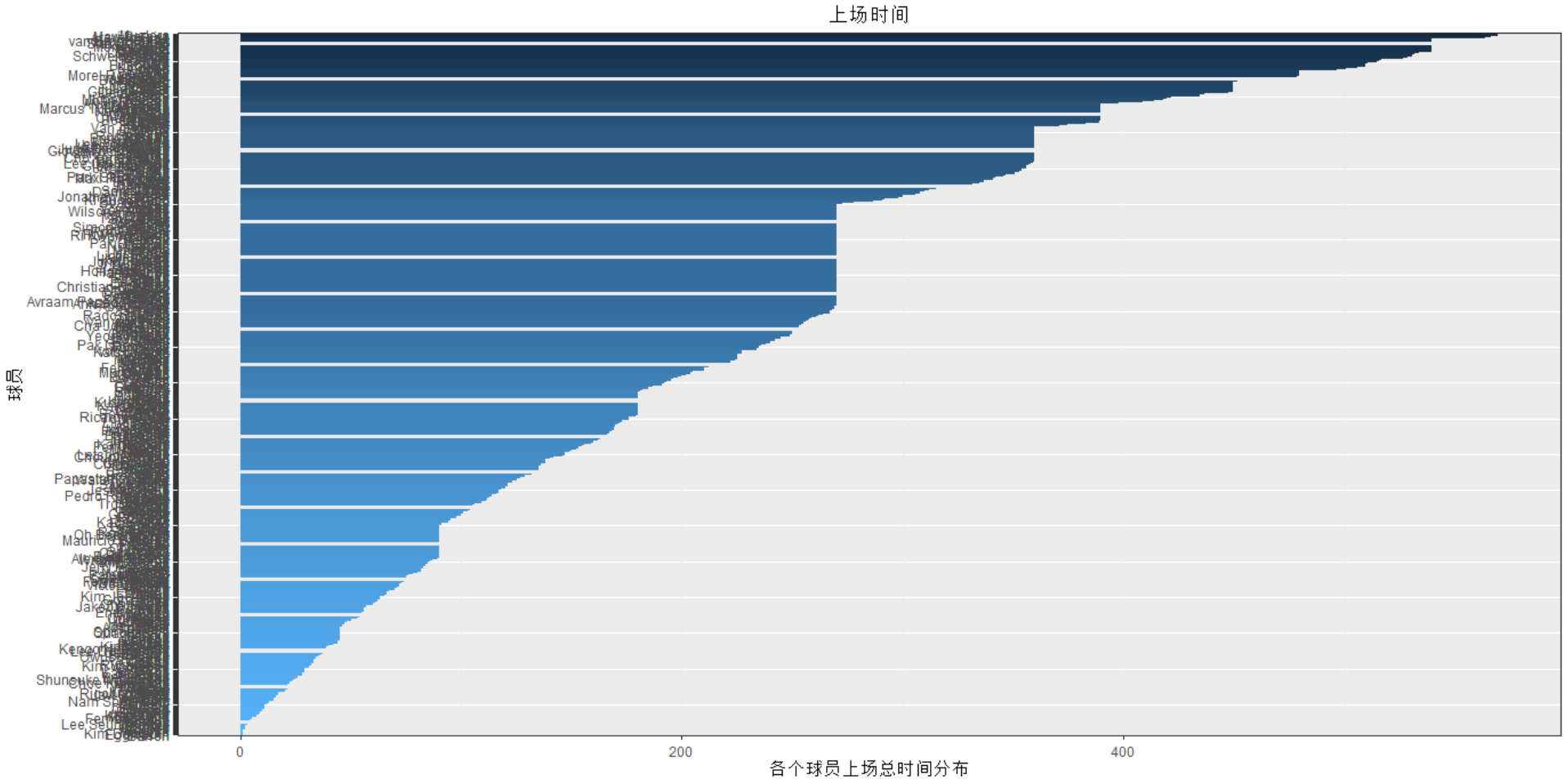12. #位置、射门、救球、传球、铲球之间的关系（散点矩阵图）

#位置、射门、救球、传球、铲球之间的关系
library(GGally)
ggpairs(world[,1:7],columns=c(2,4:7),aes(color=Position),alpha=0.8)+
theme_bw(base_family="STKaiti",base_size=10)+
theme(plot.title=element_text(hjust=0.5))+
ggtitle("散点矩阵图")


运行结果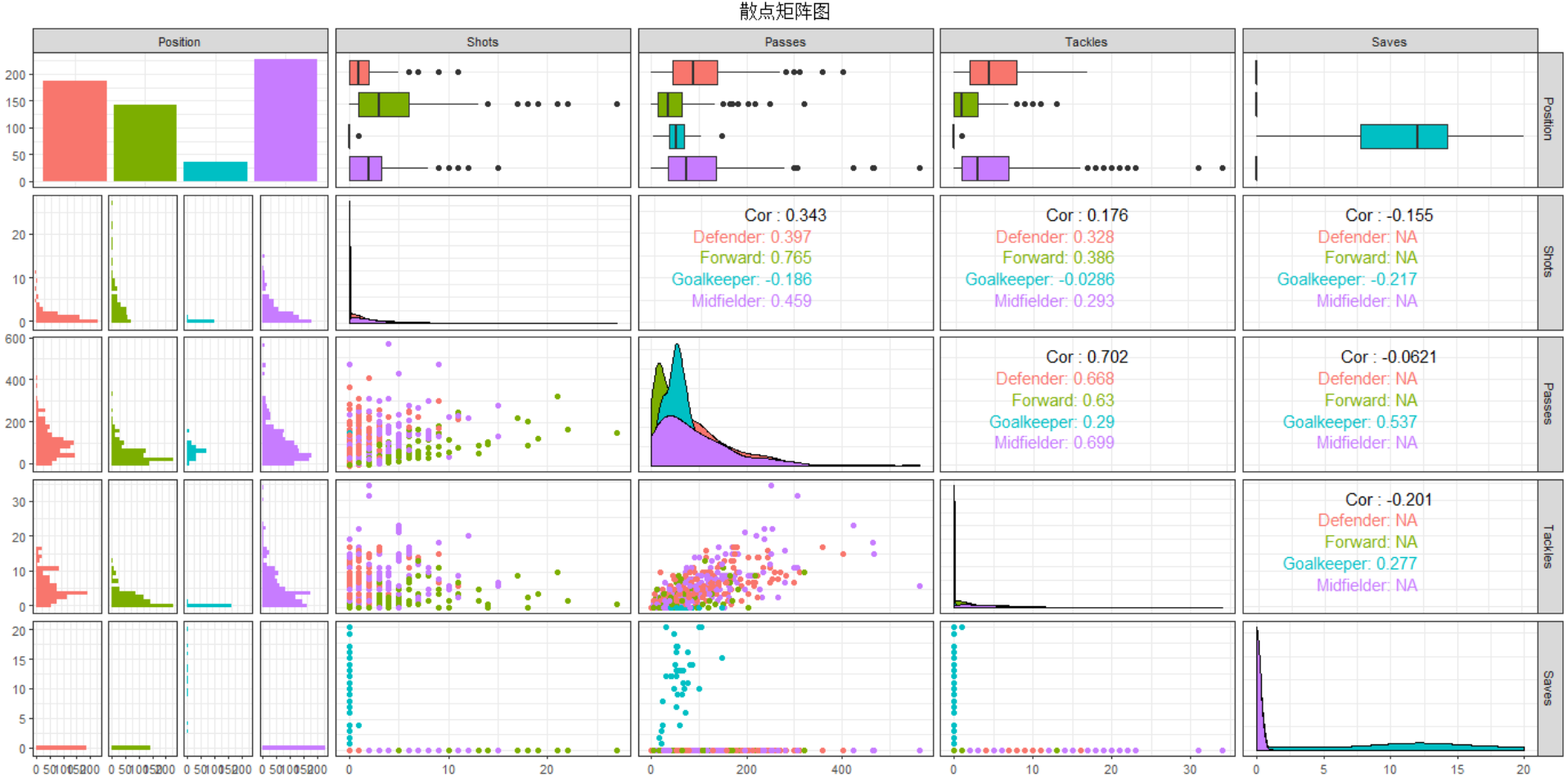由这个图可以得到许多信息。可发现后卫数量是最多的，而门卫是数量最少的。同时可看出，四个位置的传球数，射门数等都服从偏态分布。Tackles 后卫表现突出，Shots 前锋表现突出.符合常理。

展开全文r语言
• R R 原创 语言案例数据分析报告论文...R R 这个问题集的目标是让你参与到 R 中的一些活动中并且在欣赏数据可视化的重要性的同时进 这个问题集的目标是让你参与到 中的一些活动中并且在欣赏数据可视化的重要性的同时进 /
• ## R语言之数据可视化

千次阅读 2021-08-27 21:04:07
数据可视化 ggplot2包介绍 ggplot2包由Hadley Wickham编写，提供了一种基于Wilkinson所述图形语法的图形系统。ggplot2包的目标是提供一个全面的、基于语法的、连贯一致的图形生成系统，允许用户创建新颖的、有创新性...

# 数据可视化

## ggplot2包介绍

总的来说有以下几点：

• ggplot2的核心理念是将绘图与数据分离，数据相关的绘图与数据无关的绘图分离
• ggplot2保有命令式作图的调整函数，使其更具灵活性
• ggplot2将常见的统计变换融入到了绘图中。
• ggplot2是按图层作图

ggplot2图像的三个基本构成：数据、图形属性映射、几何对象

按照ggplot2的绘图理念，Plot(图)= data(数据集)+ Aesthetics(美学映射)+ Geometry(几何对象)。

例如：

# ggplot(data,aes(x=x,y=y))+geom_point()

• 数据：用于绘制图形的数据
• 映射：aes()函数是ggplot2中的映射函数, 所谓的映射即为数据集中的数据关联到相应的图形属性过程中一种对应关系, 图形的颜色，形状，分组等都可以通过通过数据集中的变量映射。
• 几何对象：我们在图中实际看到的图形元素，如点、线、多边形等。

ggplot2绘图代码如同数据公式一般，只需要套相应的公式即可绘制出丰富的图形，后续的讲解也会按照此方法。

ggplot2参考链接：

• https://ggplot2.tidyverse.org/reference/
• https://ggplot2-book.org/

ggplot2的安装方法

# install.packages("ggplot2")


## 环境配置

library(ggplot2) # 画图工具ggplot2
library(ggpubr) # 将多个图形拼接
library(plyr) # 数据处理包


### 案例数据

本文会使用到两个数据集

1.1h1n1流感问卷数据集

h1n1流感问卷数据集是关于h1n1流感问卷调查的一个数据，属于外部数据

数据集包含26,707个受访者数据，共有32个特征+1个标签（是否接种h1n1疫苗）

读取相关的数据集

h1n1_data <- read.csv("./datasets/h1n1_flu.csv", header = TRUE)


1.2波士顿房价数据集

波士顿房价数据集属于R语言自带数据集，也可以通过外部读取

读取相关的数据集

boston_data <- read.csv("./datasets/BostonHousing.csv", header = TRUE)


## 散点图

散点图是指在数理统计回归分析中，数据点在直角坐标系平面上的分布图，散点图表示因变量随自变量而变化的大致趋势，由此趋势可以选择合适的函数进行经验分布的拟合，进而找到变量之间的函数关系。

散点图的优势：

• 数据用图表来展示，显然比较直观，在工作汇报等场合能起到事半功倍的效果，让听者更容易接受，理解你所处理的数据。
• 散点图更偏向于研究型图表，能让我们发现变量之间隐藏的关系为我们决策作出重要的引导作用。
• 散点图核心的价值在于发现变量之间的关系，包括线性与非线性之间的关系。
# 读取数据
# 绘制简单的散点图 x轴选择的是lstat ,y轴选择的是medv
ggplot(data = boston_data, aes(x = lstat, y = medv)) +
geom_point()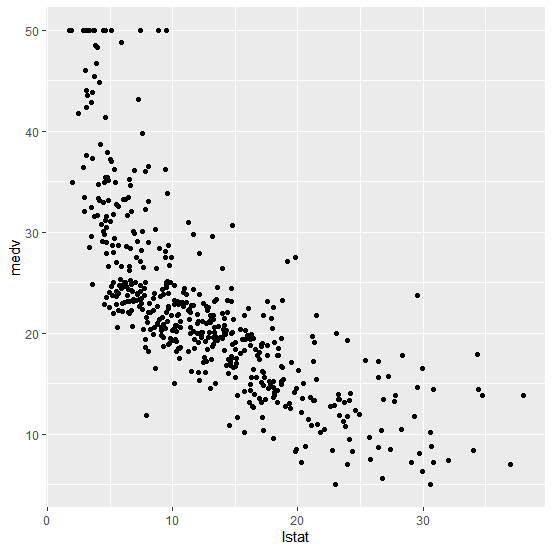上图选择的是lstat为x轴，medv为y轴绘制的散点图，x轴表示弱势群体人口所占比例，y轴表示房屋的平均价格，通过图上的数据可以看到，弱势人群的比例增加会影响房价，这2个变量呈现一定的负相关。

ggplot2可以修改散点图的性状和大小,R语言中存储了一些相关的形状。

size参数修改点的大小，color参数修改点的颜色。

# 使用第17号形状
p1 <- ggplot(data = boston_data, aes(x = lstat, y = medv)) +
geom_point(shape = 17)
# size参数修改点的大小，color参数修改点的颜色
p2 <- ggplot(data = boston_data, aes(x = lstat, y = medv)) +
geom_point(size = 3, color = "red")
ggarrange(p1, p2, nrow = 1)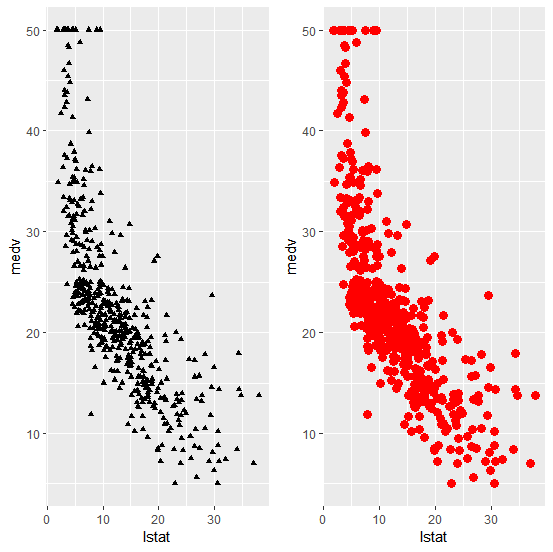可将数据集的其它属性映射到散点图的颜色属性中

p3 <- ggplot(data = boston_data, aes(x = lstat, y = medv, colour = factor(rad))) +
geom_point()
p4 <- ggplot(data = boston_data, aes(x = lstat, y = medv, colour = rad)) +
geom_point()
ggarrange(p3, p4, nrow = 1)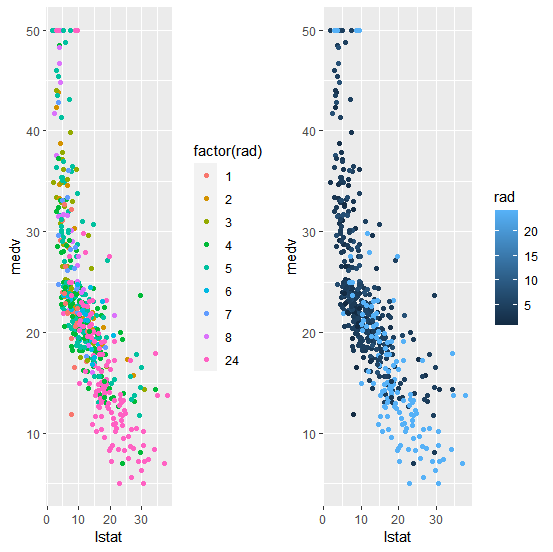ggplot2关于散点图的相关做法有很详细的介绍，相关参考链接：https://ggplot2.tidyverse.org/reference/geom_point.html

## 直方图

直方图是一种统计报告图，由一系列高度不等的纵向条纹或线段表示数据分布的情况。 一般用横轴表示数据类型，纵轴表示分布情况。
直方图可以很好的查看数据的分布情况，是常用的数据可视化展示图形。

ggplot(data = boston_data, aes(x = rad)) +
geom_histogram()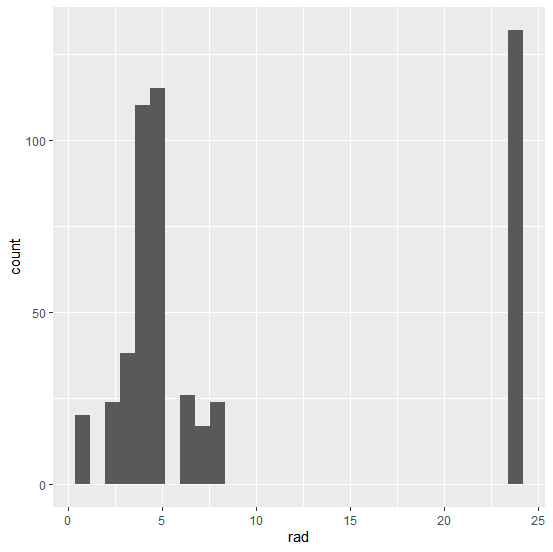可以看到ggplot2可以自动对数据进行直方图的统计

我们给直方图填充颜色，同时改变直方图类型color表示直方图的边框，fill表示直方图中的填充颜色，ggplot2支持RGB颜色表的配色方案，linetype表示直方图线的类型

RGB颜色表可以参考：http://www.mgzxzs.com/sytool/se.htm

p5 <- ggplot(data = boston_data, aes(x = rad)) +
geom_histogram(color = "black", fill = "#69b3a2")
p6 <- ggplot(data = boston_data, aes(x = rad)) +
geom_histogram(color = "black", fill = "#69b3a2", linetype = "dashed")
ggarrange(p5, p6, nrow = 1)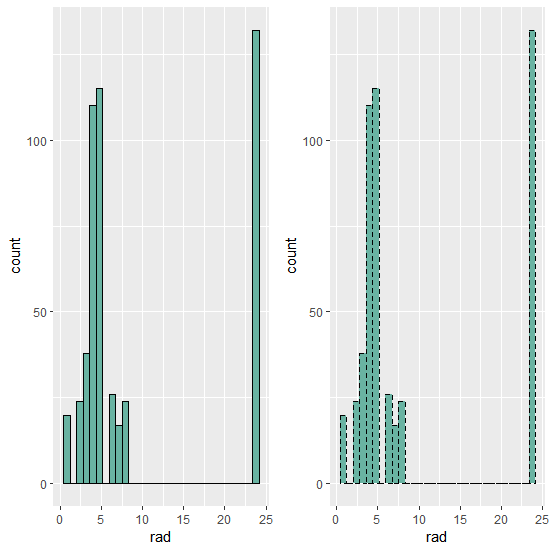ggplot2也支持在直方图上添加平均线和密度图

p7 <- p5 + geom_vline(aes(xintercept = mean(rad)), color = "blue", linetype = "dashed", size = 1)
p8 <- ggplot(data = boston_data, aes(x = rad)) +
geom_histogram(color = "black", fill = "#69b3a2", aes(y = ..density..)) +
geom_density(alpha = .2, fill = "#FF6666")
ggarrange(p7, p8, nrow = 1)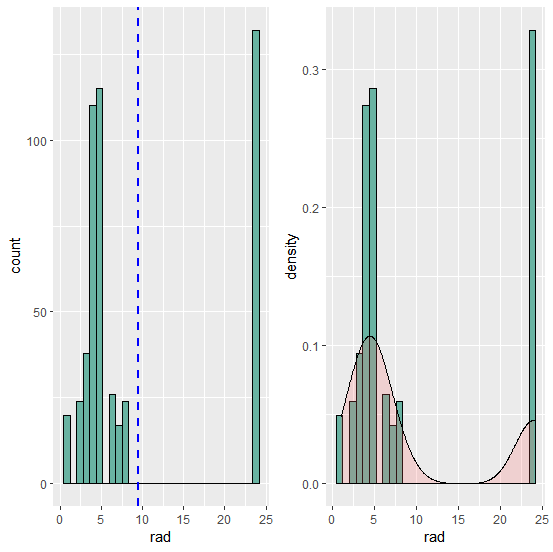ggplot2关于直方图的相关做法有很详细的介绍，相关参考链接：https://ggplot2.tidyverse.org/reference/geom_histogram.html

## 柱状图

柱状图是一种常用的数据可视化图形，根据翻译的不同，柱状图又叫长条图、柱状统计图、条状图、棒形图

柱状图图用来比较两个或以上的价值（不同时间或者不同条件），只有一个变量，通常利用于较小的数据集分析。长条图亦可横向排列，或用多维方式表达。需要注意的是柱状图与直方图是不同的数据可视化方法，不要弄混淆了。

对h1n1数据集中填写人的种族情况进行可视化展示,使用pylr包中的count对edcation进行计数统计

data <- count(h1n1_data["race"])
p <- ggplot(data, aes(x = race, y = freq)) +
geom_bar(stat = "identity")
# 也可以进行水平放置
p1 <- p + coord_flip()
ggarrange(p, p1)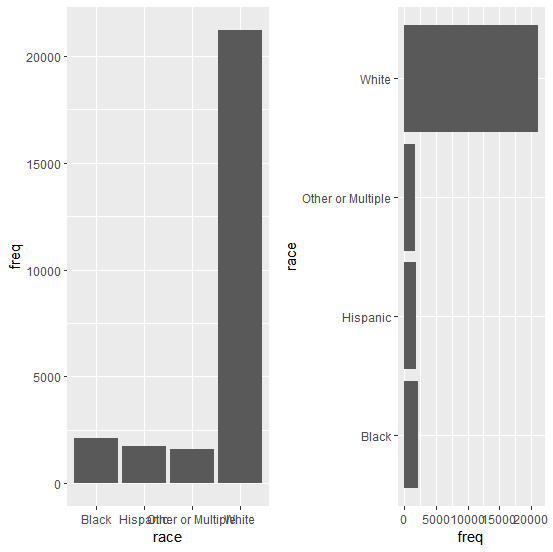可以看到左边的柱状图文字有点挡住了，我们把文字旋转45°

data <- count(h1n1_data["race"])
ggplot(data, aes(x = race, y = freq)) +
geom_bar(stat = "identity") +
theme(axis.text.x = element_text(angle = 45, hjust = 1))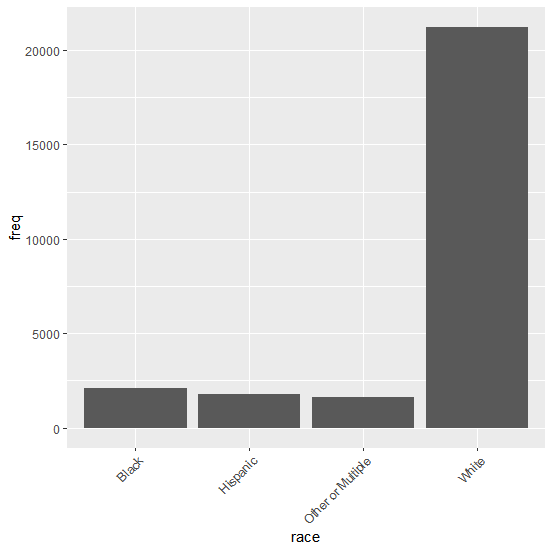对柱状图的样式进行修改

# 更改条的宽度和颜色：
# 更改条的宽度
p2 <- ggplot(data, aes(x = race, y = freq)) +
geom_bar(stat = "identity", width = 0.5) +
theme(axis.text.x = element_text(angle = 45, hjust = 1))
# 改变颜色
p3 <- ggplot(data, aes(x = race, y = freq)) +
geom_bar(stat = "identity", color = "blue", fill = "white") +
theme(axis.text.x = element_text(angle = 45, hjust = 1))
# 最小主题+蓝色填充颜色
p4 <- ggplot(data, aes(x = race, y = freq)) +
geom_bar(stat = "identity", fill = "steelblue") +
theme_minimal() +
theme(axis.text.x = element_text(angle = 45, hjust = 1))
# 选择要显示的项目
p5 <- p + scale_x_discrete(limits = c("White", "Black")) + theme(axis.text.x = element_text(angle = 45, hjust = 1))
ggarrange(p2, p3, p4, p5)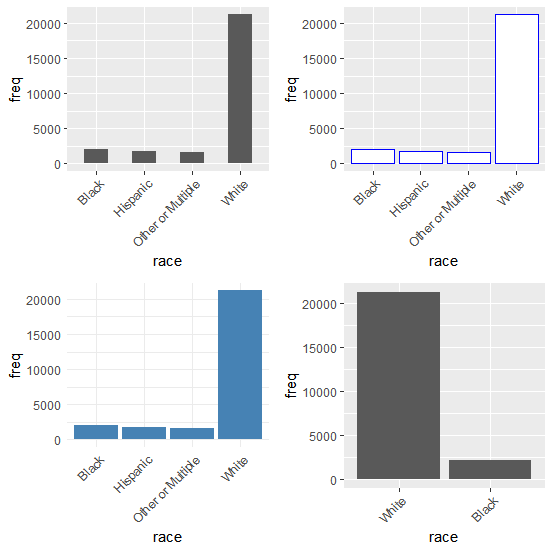对柱状图进行标签显示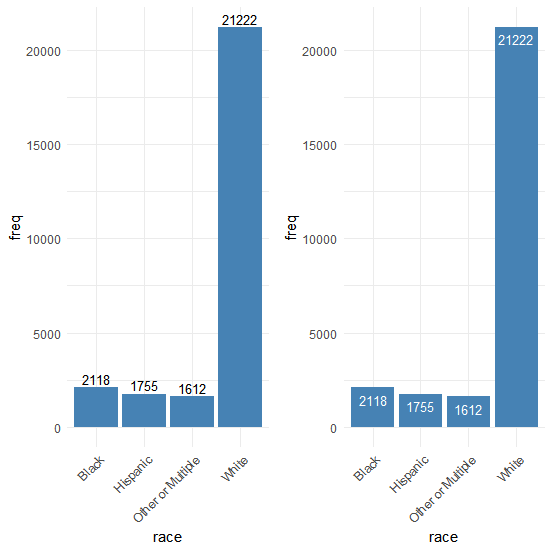如果觉得柱状图的顺序不是你想要的，可以对柱状图的顺序进行修改

data <- within(data, {
race <- factor(race, levels = c("White", "Black", "Hispanic", "Other or Multiple"))
})
ggplot(data, aes(x = race, y = freq)) +
geom_bar(stat = "identity", fill = "steelblue") +
theme(axis.text.x = element_text(angle = 45, hjust = 1))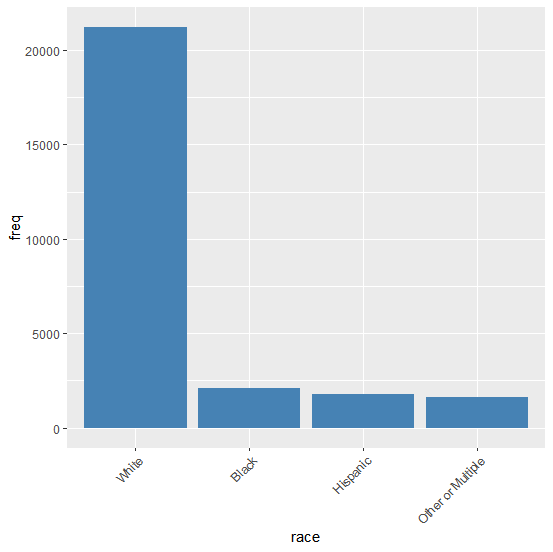ggplot2关于柱状图的相关做法有很详细的介绍，相关参考链接：
https://ggplot2.tidyverse.org/reference/geom_bar.html

## 饼状图

饼状图作为常用的数据可视化图形之一，广泛的使用在各个领域，能够很清楚展示数据的所占的百分比。
ggplot2并没有类似于geom_pie()这样的函数实现饼图的绘制，但ggplot2有一个理念，就是通过极坐标变换绘制饼图

饼图在ggplot2中就是通过极坐标变换获得，在绘制饼图之前需要绘制堆叠的条形图，通过将条形图进行极坐标变换后，就能实现饼图绘制了。

对h1n1问卷表中race数据进行数据展示

data <- count(h1n1_data["race"])
ggplot(data = data, aes(x = "", y = freq, fill = race)) +
geom_bar(stat = "identity")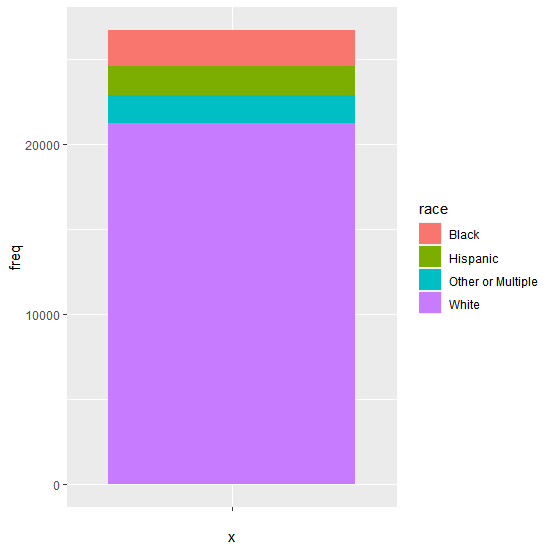堆叠的条形图绘制完后，接下来就需要进行极坐标变换了，ggplot2中coord_polar()函数可以非常方便的实现极坐标变换。

ggplot(data = data, aes(x = "", y = freq, fill = race)) +
geom_bar(stat = "identity") +
coord_polar(theta = "y")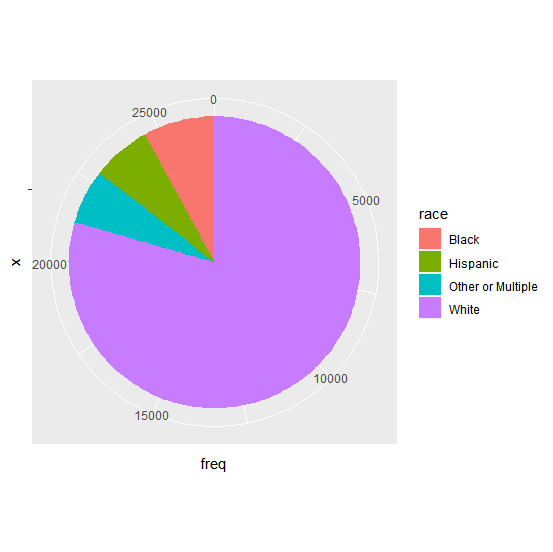看起来像饼图了，但是饼图周围还有多余的数字，如何清除呢？
这里的标签其实就是坐标轴的标签，可以通过labs()函数将其清除。

ggplot(data = data, aes(x = "", y = freq, fill = race)) +
geom_bar(stat = "identity") +
coord_polar(theta = "y") +
labs(x = "", y = "", title = "") +
theme(axis.text = element_blank())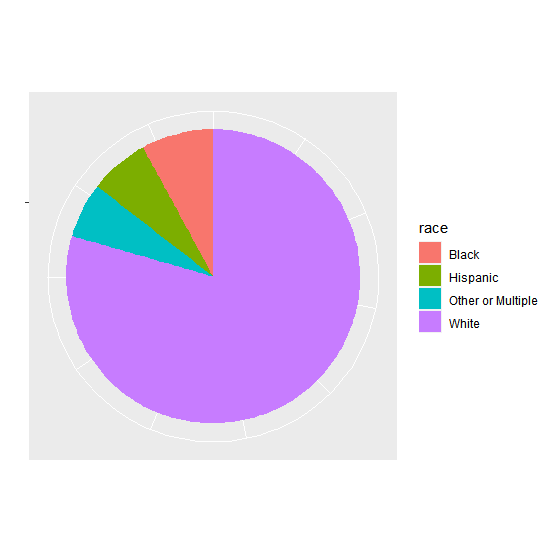接下来就是显示各个所占的比例
第一种方法，将百分比直接显示在图例中，这种方式适合分类较多的情况。

label_value <- paste("(", round(data$freq / sum(data$freq) * 100, 1), "%)", sep = "")
label_value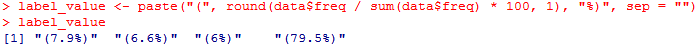将计算的百分比和race匹配

label <- paste(data\$race, label_value, sep = "")
label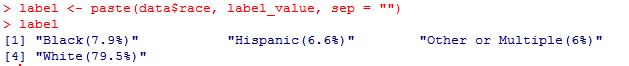接下来就是将这些百分比标签放到图例中

ggplot(data = data, aes(x = "", y = freq, fill = race)) +
geom_bar(stat = "identity") +
coord_polar(theta = "y") +
labs(x = "", y = "", title = "") +
theme(axis.text = element_blank()) +
scale_fill_discrete(labels = label)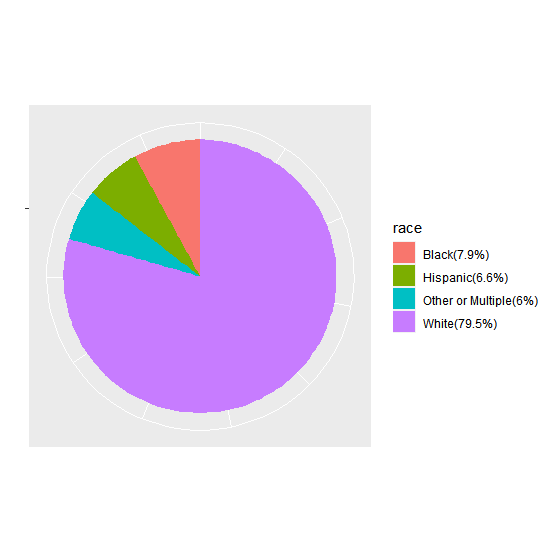第二种方法，直接将百分比放到各自的饼区中。

首先是去掉饼图中的图例

ggplot(data = data, aes(x = "", y = freq, fill = race)) +
geom_bar(stat = "identity") +
coord_polar(theta = "y") +
labs(x = "", y = "", title = "") +
theme(axis.text = element_blank()) +
theme(legend.position = "none")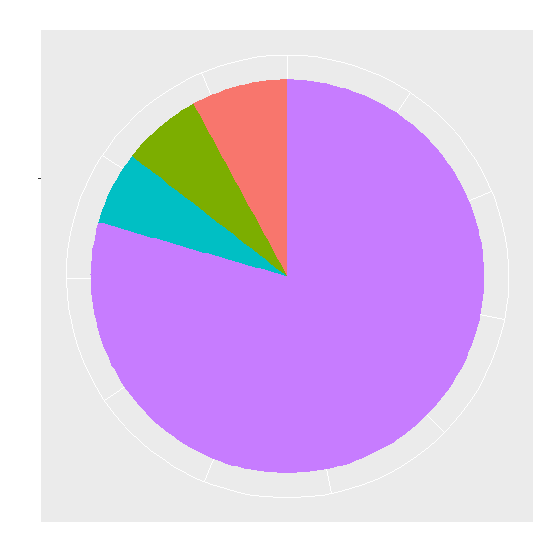将标签放置在饼图中

ggplot(data = data, aes(x = "", y = freq, fill = race)) +
geom_bar(stat = "identity", width = 1) +
coord_polar(theta = "y") +
labs(x = "", y = "", title = "") +
theme(axis.text = element_blank(), legend.position = "none") +
geom_text(aes(label = label), size = 3, position = position_stack(vjust = 0.5))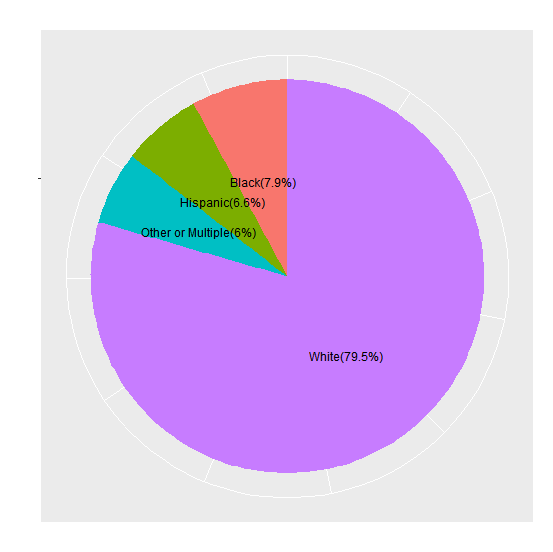## 折线图

折线图作为反映数据变化的趋势是常用的数据可视化图形之一，在ggplot2中通过geom_line()这个函数进行绘制。

data <- count(boston_data["rad"])
data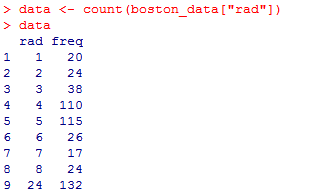data <- data[1:8, ]
ggplot(data, aes(x = rad, y = freq)) +
geom_line()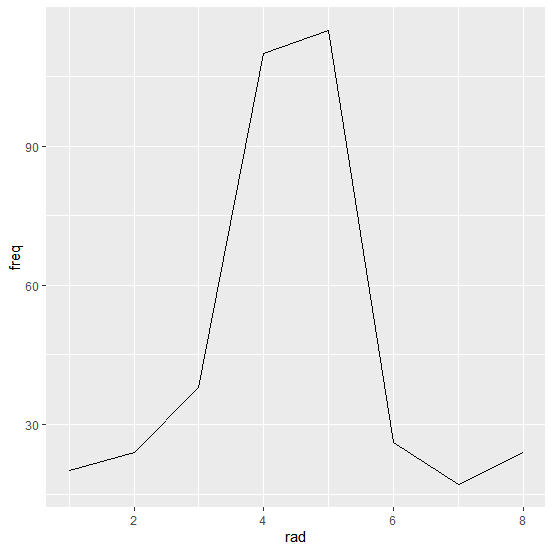有时候我们需要在折线图上显示对应x轴的点数据，从而可以更加清晰的辨别原始数据,这特别适合数据比较稀疏的情况

ggplot(data, aes(x = rad, y = freq)) +
geom_line() +
geom_point(size = 4)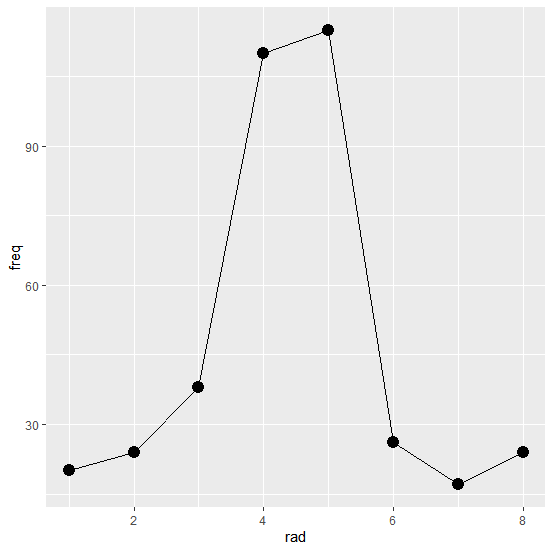我们调整横坐标的显示刻度

ggplot(data, aes(x = rad, y = freq)) +
geom_line() +
geom_point(size = 4) +
scale_x_continuous(breaks = c(1:8))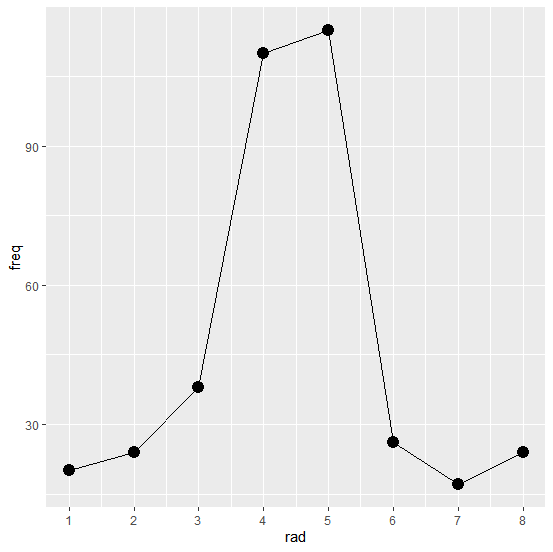也可以修改线的类型和颜色

ggplot(data, aes(x = rad, y = freq)) +
geom_line(linetype = "dashed", color = "red") +
geom_point(size = 4) +
scale_x_continuous(breaks = c(1:8))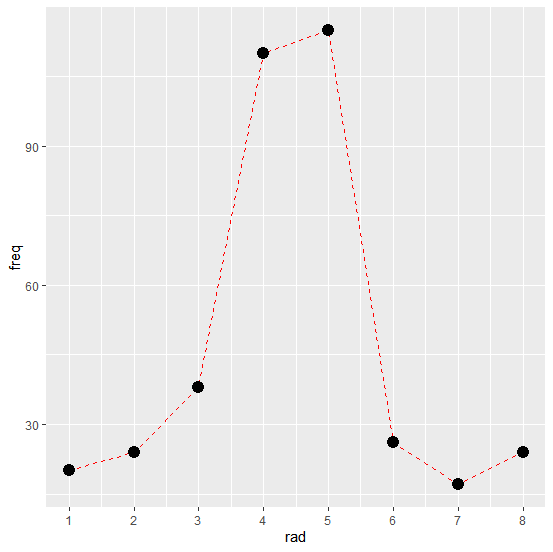ggplt2关于折线图的相关做法的参考链接：
https://ggplot2.tidyverse.org/reference/geom_abline.html

## ggplot2扩展包主题

R语言中的ggplot2包里面的风格固定，在需要特殊的图形时，需要更改甚至自定义设置主题。
ggplot2内置了8种风格的主题

主题函数效果
theme_bw()网格白色主题
theme_classic()经典主题
theme_dark()暗色主题，可用于对比
theme_gray()默认主题
theme_light()浅色坐标带网格
theme_linedraw()黑色网格线
theme_minimal()极简主题
theme_void()空白主题

我们来试一试不同的主题

p <- ggplot(data = boston_data, aes(x = lstat, y = medv, colour = rad)) +
geom_point()
p1 <- p + theme_bw() + labs(title = "网格白色主题") + theme(legend.position = "none")
p2 <- p + theme_classic() + labs(title = "经典主题") + theme(legend.position = "none")
p3 <- p + theme_dark() + labs(title = "暗色主题") + theme(legend.position = "none")
p4 <- p + theme_gray() + labs(title = "默认主题") + theme(legend.position = "none")
p5 <- p + theme_light() + labs(title = "浅色坐标带网格") + theme(legend.position = "none")
p6 <- p + theme_linedraw() + labs(title = "黑色网格线") + theme(legend.position = "none")
p7 <- p + theme_minimal() + labs(title = "极简主题") + theme(legend.position = "none")
p8 <- p + theme_void() + labs(title = "空白主题") + theme(legend.position = "none")
ggarrange(p1, p2, p3, p4, p5, p6, p7, p8, ncol = 4, nrow = 2, heights = 1.2)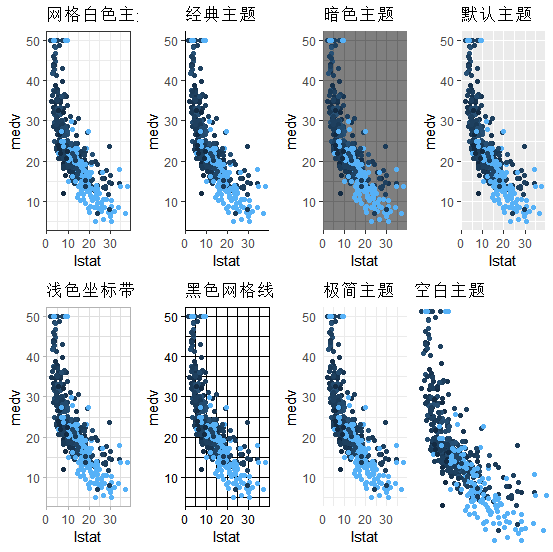除了ggplot2自带的主题外，还有许多拓展主题包，比如：ggthemes、ggthemr

ggthemes相关链接：https://github.com/jrnold/ggthemes

ggthemr相关链接：https://github.com/Mikata-Project/ggthemr

因为ggthemr没有上cran，因此需要通过github安装

# devtools::install_github('Mikata-Project/ggthemr')


greyscale主题方案

library(ggthemr)
ggthemr("greyscale")
p3 <- ggplot(data = boston_data, aes(x = lstat, y = medv, colour = factor(rad))) +
geom_point()
p4 <- ggplot(data = boston_data, aes(x = lstat, y = medv, colour = rad)) +
geom_point()
ggarrange(p3, p4, nrow = 1)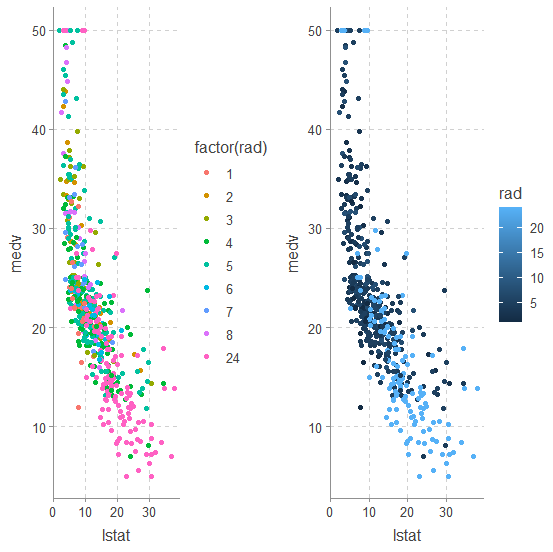light主题，配色非常的温柔

library(ggthemr)
ggthemr("light")
p3 <- ggplot(data = boston_data, aes(x = lstat, y = medv, colour = factor(rad))) +
geom_point()
p4 <- ggplot(data = boston_data, aes(x = lstat, y = medv, colour = rad)) +
geom_point()
ggarrange(p3, p4, nrow = 1)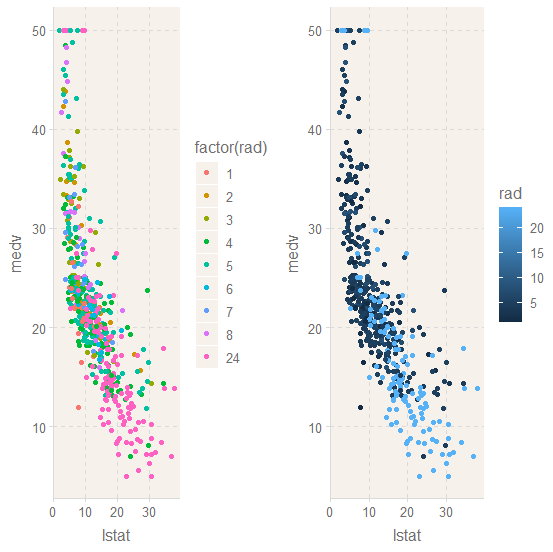# 感谢Datawhale对开源学习的贡献！

展开全文r语言 人工智能
• 基于R语言的汽车消费数据挖掘及可视化方法.pdf
• R具有强大的统计计算功能和便捷的数据可视化系统。《R数据可视化手册》重点讲解R的绘图系统，指导读者..., 《R数据可视化手册》是学习R中丰富的数据可视化方法的权威手册，非常适合对R 语言有基本的了解的读者阅读。
• 梳理总结了R语言8种数据可视化案例，涵盖了基本及高级绘图工具及函数，方便后来者学习。
• 收集了2004年（第一届）至今中（全）国研究生数学建模竞赛获奖数据，并基于R语言，进行了数据处理和可视化的工作。 这项工作最初的起因是，作者和队友在2015、2016、2017年参赛并获奖后，好奇一共有多少人连续获奖...R
• 今天给大家介绍一个超级酷的R包{TSstudio}[1,2]，可用于时间序列分析(timeseriesanalysis)作图，里面包含很多实用的函数，其中一些还可兼容多种数据形式(如timeseries数据,data.frame,tibble,data.table等等)。...

## 前言

今天给大家介绍一个超级酷的R包{TSstudio}[1,2]，可用于时间序列分析(time series analysis)作图，里面包含很多实用的函数，其中一些还可兼容多种数据形式(如time series数据, data.frame, tibble, data.table等等)。

首先，安装与载入用到的R包。

install.packages("TSstudio")
install.packages("ggplot2")
library(TSstudio) # 数据 USgas/ 作图
library(ggplot2)  # 数据 economics

这次作图用到的数据有两个："USgas"和"economics"。

我们查看一下"USgas"的情况：

# 数据类型 Time series data
data("USgas")
ts_info(USgas)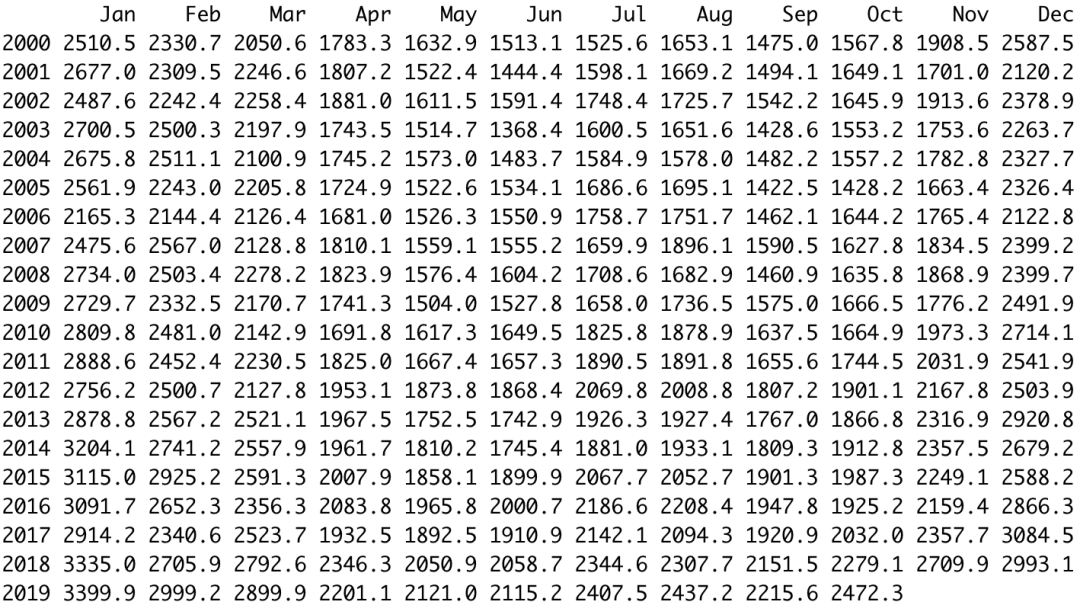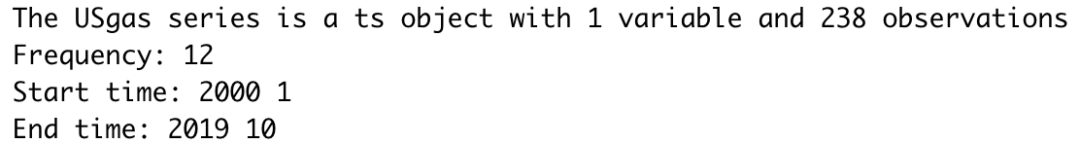上述数据为time series数据，数据从2000年1月开始到2019年10月终止，每个月一次。

数据集"economics"为tibble格式，也查

展开全文r语言
• 空间数据可视化&地图绘制&R语言可复现 绘制地理空间数据是一项常见的可视化任务，需要专门的工具。通常，问题可以分解为两个问题： 使用一个数据源绘制地图 将来自另一个信息源的元数据添加到地图中。 有...r语言
• 目标 本文的目的是对如何在R中进行生存分析进行简短而全面的评估。关于该主题的文献很广泛，仅涉及有限数量的（常见）问题...以下是本次审查中用于读取，管理，分析和显示数据的软件包。 运行以下行以安装和加载...R语言 生存分析
• R语言一键批量完成差异统计和可视化 gggenes绘制多物种基因结构比较 数据可视化完美指南-R-python Pathview包：整合表达谱数据可视化KEGG通路 Matplotlib绘制的27个常用图(附对应代码实现) 让你的单细胞数据动起来...人工智能 编程语言
• 书中有更多的R语言数据可视化分析使用案例，可学习更多的关于R语言数据可视化的内容。 数据可视化，是关于数据视觉表现形式的科学技术研究，它旨在借助图形化手段，清晰有效地传达与沟通信息，是科学可视化与信息...r语言 大数据 数据挖掘
• R语言使用案例一：设计函数：自动获取股票数据可视化。封装的函数功能包括：1.使用quantmod包中的getSymbols函数来抓取实时的股票数据;2.使用quantmod包中的chartSeries函数进行可视化输出K线图和相关指标;3.利用DT......